Name:    Practice Final A

Multiple Choice
Identify the choice that best completes the statement or answers the question.

1.

What is E0 for the cell utilizing the following half-reactions::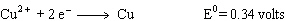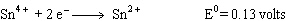A) -0.47 volts B) 0.47 volts C) 0.21 volts D) -0.21 volts

2.

Which of the following processes results in a decrease in entropy?
 A) NH3 (l), –35 °C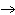NH3 (g), –35 °C B) O2 (g) , 300 KO2 (g), 400 K C) N2 (g), 25 °CN2 (aq), 25 °C D) H2O (s), 0 °CH2O (l), 0 °C

3.

In the voltaic cell shown below, which reaction occurs at the cathode?

Cu | Cu2+ ||  Ag+ | Ag
 A)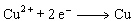B)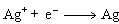C)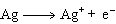D)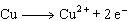4.

Which of the following conditions is sponatneous only at high temperatures?
 A)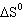> 0 ,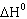> 0 B)< 0 ,< 0 C)< 0 ,> 0 D)> 0 ,< 0

5.

Calculate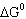for the reaction: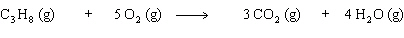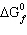(kJ/mol) –24.5 0 –394.4 –228.6

 A) 598.5 kJ B) –598.5 kJ C) –2073.1 kJ D) 2073.1 kJ

6.

Which of the following statements is true for the electrochemical cells?
 A) The free energy change (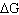) is negative for the voltaic cell. B) Oxidation occurs at the cathode in the voltaic cell. C) The current flows from cathode to anode in both cells. D) The free energy change () is negative for the electrolytic cell.

7.

Which letter represents the cathode in the cell notation shown below:

P | Q ||  R | S
 A) P B) Q C) S D) R

8.

The voltaic cell made up of copper and metal M has E0 = 0.62 V.  If E0Cu = 0.34 V, what is E0M?
Cu2+ (aq) +  M (s)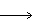Cu (s) + M2+ (aq)
 A) –0.28 V B) –0.96 V C) 0.96 V D) 0.28 V

9.

Predict the sign offor the following reaction:

Pb (s) + Cl2 (g)PbCl2 (s)
 A)= 0 B)< 0 C)> 0 D) More information is needed to make a reasonable prediction

10.

Calculatefor the reaction shown below: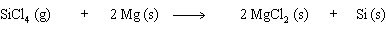S° (J/Kmol) 330.73 32.68 89.62 18.83

 A) –198.02 J/K B) –330.73 J/K C) –254.96 J/K D) 254.96 J/K J/K

11.

Calculate E0cell and indicate whether the overall reaction shown is spontaneous or non-spontaneous.
2 Cr (s)  +  3 I2 (s)2 Cr3+ (aq) + 6 I (aq)
 A) E0cell = –1.28 V, non-spontaneous B) E0cell = –1.28 V, spontaneous C) E0cell = 1.28 V, non-spontaneous D) E0cell = 1.28 V, spontaneous

12.

Hydrogen sulfide decomposes as shown below: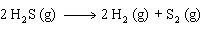For this reaction298 = 78.1 J/K,298 = 169.4 kJ, and298 = 146.1 kJ.  What is the value of900?
 A) 240 kJ B) 48.4 kJ C) 99.1 kJ D) –70100 kJ

13.

Based on the reduction potentials given, which of the following substances is the strongest reducing agent?

A2+ (aq)  +  2 eA (s)        E0 = –0.365 V
B2+ (aq)  +  2 eB (s)        E0 = –2.868 V
C2+ (aq)  +  2 eC (s)        E0 = 1.18 V
D2 (l)  +  2 e2 D (aq)        E0 = 1.066 V
 A) A B) B C) C D) D

14.

The temperature at which the equilibrium below is established at 1 atm is the normal melting point of phosphoric acid: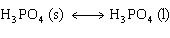Use the following data to determine this temperature.

 Substance H3PO4 (s) H3PO4 (l)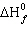(kJ/mol) –1284.4 –1271.7(kJ/mol) –1124.3 –1123.6 S° (J/K mol) 110.5 150.8
 A) 347 K B) 286 K C) 305 K D) 315 K

15.

Which of the following species can act both as an oxidizing and a reducing agent?
 A) I2 B) NO3- C) SO42- D) S2-